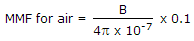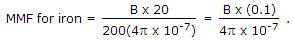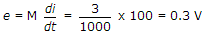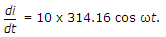# Electronics and Communication Engineering - Networks Analysis and Synthesis

1.

A magnetic circuit has an iron length of 20 cm and air gap length 0.1 cm. If μr = 200 then

 A. mmf for iron and air gap are equal B. mmf for iron is much less than that for air gap C. mmf for iron is much more than that for air gap D. either (a) or (c)

Explanation:2.

A circuit is fed by two sources of the same frequency and values 100 ∠0° V and 20 ∠30°V. The current in a particular branch is 5 ∠19° A. If the magnitudes of the sources and changed to 200 ∠0° V and 40 ∠30° V, the current in that branch should be

 A. 5 ∠19° A B. 10 ∠19° A C. 10 ∠38° A D. 10 ∠9.5° A

Explanation:

Since strengths of both sources are doubled, the current would become twice.

3.

Two coils X and Y have self inductances of 5 mH and 10 mH and mutual inductance of 3 mH. If the current in coils X change at a steady rate of 100 A/s, the emf induced in coil Y is

 A. 0.3 V B. 0.5 V C. 1 V D. 1.5 V

Explanation:4.

Two capacitors of 2 μF and 4 μF capacitance are connected in series across a 30 V dc battery. After the capacitors have been charged, the voltage across them will be

 A. 15 V each B. 10 V and 20 V C. 20 V and 10 V D. 30 V each

Explanation:

Charge will be the same. Since Q = C V voltage will be inversely proportional to Q. Hence 20 V and 10 V.

5.

A 50 Hz current has an amplitude of 10 A. The rate of change of current at t = 0.005 after i = 0 and is increasing is

 A. 2221.44 A/s B. 0 C. - 2221.44 A/s D. - 3141.6 A/s

i = 10 sin 314.16 t,At t = 0.005, i = 314.16 cos (314.16 x .005) = 0.